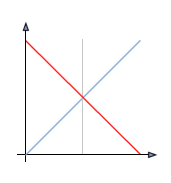# Image processing

## Introduction to image processing

The set of techniques allowing a digital image to be modified with the aim of improving it or of extracting information from it, is known as digital image processing.

## Histogram

A histogram is a statistical graph that allows the intensity distribution of the pixels of an image, i.e. the number of pixels for each luminous intensity, to be represented. By convention, a histogram represents the intensity level using X-coordinates going from the darkest (on the left) to lightest (on the right).

Thus, the histogram of an image with 256 levels of grey will be represented by a graph having 256 values on the X-axis and the number of image pixels on the Y-axis. Let us consider, for example, the following image made up of levels of grey:The histogram and the palette associated with this image are, respectively, the following: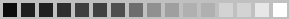The histogram reveals that there are many more light grey tones present in the image than dark grey tones.
The tone of grey that is most used is the 11th from the left.

Several histograms are necessary for color images. For example, for an image coded in RGB there are:

• a histogram representing the luminance distribution,
• three histograms representing the distribution of the values of the red, blue and green components respectively.

## Cumulative histogram

The cumulative histogram represents the cumulative intensity distribution of the pixels of an image, i.e. the number of pixels having at least a given luminous intensity.

## Modification of the histogram

A histogram is a very useful tool to study the distribution of the components of an image but it also allows the contrast and the range of colors for over-exposed or under-exposed images to be corrected. Moreover, its modification does not deteriorate the information contained in the image but makes it more or less visible.

The modification of a histogram is usually represented on a curve (called a tonal curve) indicating the total modification of the image components with the initial values on the X-axis and the values after the modification on the Y-axis. The tonal curve corresponds to a transfer function defined by a translation table called a look up table, which is written LUT. Thus, the diagonal indicates the curve along which the initial values are equal to the end values, i.e. when no modification has taken place. The values on the left of the mean value on the x-axis represent “light” pixels while those on the right represent dark pixels.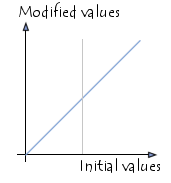Thus, if the histogram modification curve is located below the diagonal, the pixels will have lower values and will thus have been lightened. On the contrary, if the curve is above the diagonal, the pixels will have been darkened.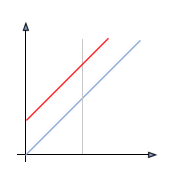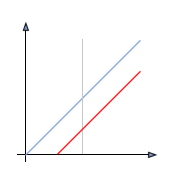Lightening Darkening

## Histogram equalization

The purpose of histogram equalization is to harmonize the image luminosity level distribution, in such a way that each of the histogram levels tend toward containing the same number of pixels. This operation aims at increasing the image nuances.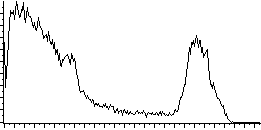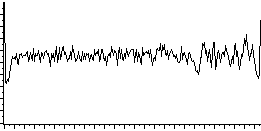The tonal curve corresponding to the histogram equalization depends completely on the image. However most applications usually offer a tool that makes it possible to carry out this operation automatically.

## Histogram stretching

Histogram stretching (also called "histogram linearization" or "dynamic range expansion") consists in distributing the pixel appearance frequencies over the entire width of the histogram. Thus, it is an operation that consists in modifying the histogram in such a way as to distribute the intensities on the scale of values available as well as possible. This amounts to extending the histogram so that the value of the lowest intensity is zero and that of the highest is the maximum value.

In this way, if the values of the histogram are very close to each other, the stretching will make it possible to provide a better distribution in order to make light pixels even lighter and dark pixels closer to black.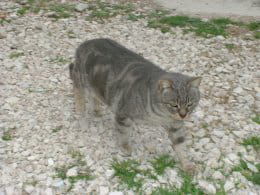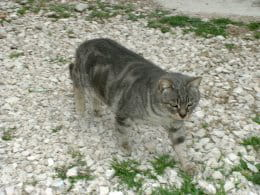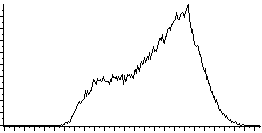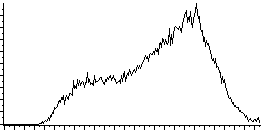It is thus possible to increase the contrast of an image. For example, an image that is too dark will become more “visible”. However this does not always yield good results.

The tonal curve corresponding to the spreading out of the histogram is as follows: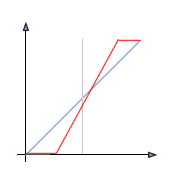## Thresholding

The operation known as “simple thresholding” consists in using zero for all pixels whose level of grey is below a certain value (called the threshold) and the maximum value for all the pixels with a higher value. Thus, the result of the thresholding is a binary image containing black and white pixels; this is why the term binarization is sometimes used. Thresholding makes it possible to highlight forms or objects in an image. However the difficulty lies in the choice of the threshold to use.

The following is an image with 256 levels of grey and the result of a thresholding operation with threshold values of 125 and 200 respectively: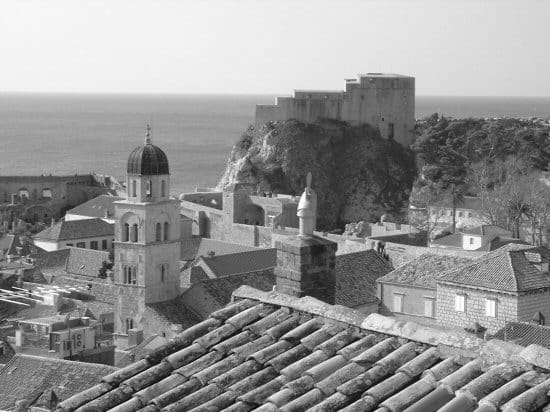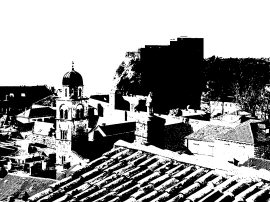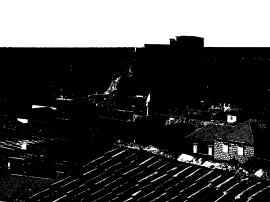The tonal curve of the thresholding operation is the following: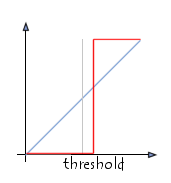As opposed to “simple thresholding”, it is possible to define two threshold values, which are the lower and upper limits respectively, in order to use the maximum value for pixels having a value between these limits and to use zero for all other values: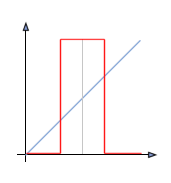## Inversion (negative)

The inversion operation consists in reversing the values of the pixels relative to the average of the possible values, as its name says. The result obtained is called the negative.The tonal curve of the video inversion operation is the following: